# ISEE Middle Level Math : How to find the distributive property

## Example Questions

### Example Question #11 : How To Find The Distributive Property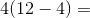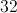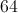Explanation:

First complete the subtraction inside the parentheses, and then multiply: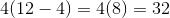### Example Question #12 : How To Find The Distributive Property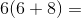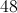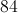Explanation:

First complete the addition inside the parentheses, and then multiply the result by the constant outside of the parentheses: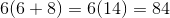### Example Question #12 : How To Find The Distributive Property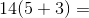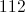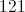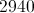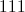Explanation:

First complete the addition inside of the parentheses, then multiply by the constant outside of the parentheses: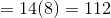### Example Question #11 : How To Find The Distributive Property

Simplify the expression: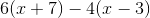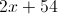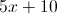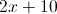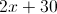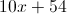Explanation:

Distribute, then collect like terms, making sure you switch the symbols in the second disribution due to the minus: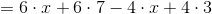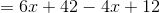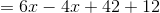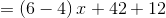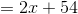### Example Question #12 : How To Find The Distributive Property

Simplify: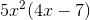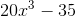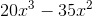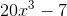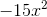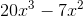Explanation: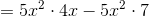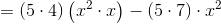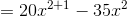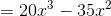### Example Question #13 : How To Find The Distributive Property

Simplify: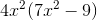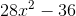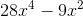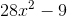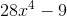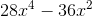Explanation: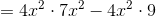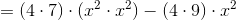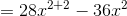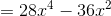### Example Question #582 : Numbers And Operations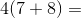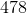Explanation:

Using the distributive property, multiply: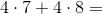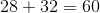Answer:### Example Question #18 : How To Find The Distributive Property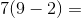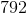Explanation:

Using the distributive property, multiply: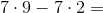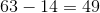Answer:### Example Question #11 : Distributive Property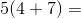Explanation:

Using the distributive property, multiply: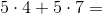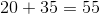Answer:### Example Question #14 : How To Find The Distributive Property

Use distributive property to write out: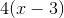Not enough information provided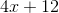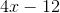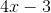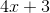Explanation:Any time a number is next to a variable that means we need to multiply. Keep in mind this is the distributive property, so we must multiply everything in the parentheses by the number next to it.

In this case we must multiplybyandbyThis gives us.

This is our answer because we are only asked to use distributive property to write this out.

### All ISEE Middle Level Math Resources